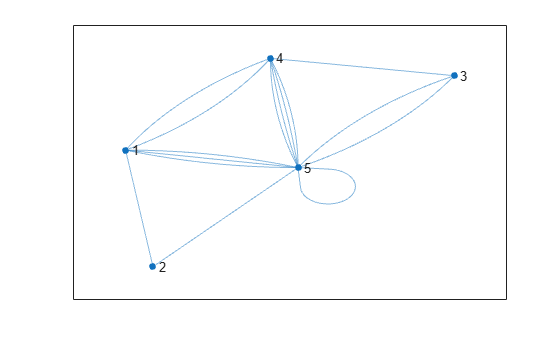Documentation

# ismultigraph

Determine whether graph has multiple edges

## Syntax

``tf = ismultigraph(G)``

## Description

example

````tf = ismultigraph(G)` returns logical `1` (`true`) if `G` has multiple edges between any two nodes. Otherwise, it returns logical `0` (`false`).By convention, `ismultigraph` returns logical `0` (`false`) for graphs that contain self-loops, but no repeated edges. However, a graph with multiple self-loops is considered to be a multigraph.```

## Examples

collapse all

Use `ismultigraph` to determine whether an input graph has multiple edges between two nodes.

Create a graph.

```G = graph([1 1 1 1 1 2 2 2],[2 2 3 4 5 6 7 8]); plot(G)```Check to see if `G` is a multigraph. The result is logical `1` (`true`) because there are two edges between nodes 1 and 2.

`tf = ismultigraph(G)`
```tf = logical 1 ```

Use `ismultigraph` to determine whether a graph needs to be simplified.

It is common to encounter duplicate edges when you create an empty graph and programmatically add edges to it with `addedge`. The data used for the edges needs to be unique to avoid duplicates.

To demonstrate this, create an empty graph and a matrix with two columns of random numbers. Since the random numbers are only between 1 and 5, this data produces multiple edges.

```G = graph; rng default % for reproducibility X = randi(5,15,2)```
```X = 15×2 5 1 5 3 1 5 5 4 4 5 1 4 2 1 3 5 5 5 5 4 ⋮ ```

Instead of cleaning the source data to ensure the rows are unique, add all of the edges to the graph. Plot the graph for reference.

```G = addedge(G,X(:,1),X(:,2)); plot(G)```Test to see if the graph is a multigraph and, if it is, use `simplify` to remove repeated edges and self-loops.

```if ismultigraph(G) G = simplify(G); end```

Plot the resulting graph.

`plot(G)`## Input Arguments

collapse all

Input graph, specified as either a `graph` or `digraph` object. Use `graph` to create an undirected graph or `digraph` to create a directed graph.

Example: `G = graph(1,2)`

Example: `G = digraph([1 2],[2 3])`